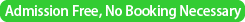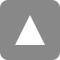﻿ 第41回統計地震学セミナー / The 41st Statistical Seismology Seminar | 統計数理研究所

# 第41回統計地震学セミナー / The 41st Statistical Seismology Seminar

(Date&Time)
2014年3月4日（火）
/ 4 March, 2014 (Tuesday)　15:00-17:30(Place)

/ Room D312B @ Institute of Statistical Mathematicsプログラム
(Program)

15:00 –
Distributions on Torus, Cylinder and Disc
By Wang, Min-Zhen (Institute of Statistical Mathematics)

【Abstracts】WANG, Min-Zhen and SHIMIZU, Kunio
Statistics for data which include angular observations is known as directional statistics. Bivariate circular data such as wind directions measured at two points in time are modeled by using bivariate circular distributions or distributions on the torus. Likewise circular-linear data are modeled by using distributions on the cylinder and disc. We propose some extensions of distributions on the torus, cylinder and disc in the framework of directional statistics.　 A new circular distribution (Wang and Shimizu, 2012) is also introduced, which is obtained by applying the Mӧbius transformation to a univariate cardioid random variable. The distribution function, trigonometric moments, and conditions for unimodality and symmetry are studied. Kato and Jones (2010) study a family of distributions which is obtained by applying the Mӧbius transformation to a von Mises random variable, and we discuss the relationship between our model and the Kato--Jones model. The bivariate circular case (Wang and Shimizu, 2012) which is generated from a circular-circular structural model linked with Mӧbius transformation or a method of trivariate reduction. The joint probability density function,  trigonometric moments and circular-circular correlation coefficient are explicitly expressed. An illustration is given for wind direction data at 6 a.m. and noon as an application of the bivariate cardioid distribution. The distributions on the cylinder we proposed is generated from a combination of von Mises and transformed Kumaraswamy distributions. It is an extension of the Johnson and Wehrly (1978) model.
The marginal and conditional distributions of the proposed distribution are given. A distribution using the method of generating a cylindrical distribution with specified marginals is also proposed. We generate skew or asymmetric distributions on the disc by using the Mӧbius transformation and modified Mӧbius transformations as extensions of the Mӧbius distribution proposed by Jones (2004). The new distributions called the modified Mӧbius distributions have six parameters. They can be reduced to the Mӧbius and uniform distributions as special cases, but many members of the family are skew distributions for both the linear and the angular random variables. Some properties such as the joint probability and marginal density functions of the proposed distributions are obtained.

References:
 Johnson, R. A. and Wehrly, T. E. (1978). Some angular-linear distributions and related regression models. Journal of the American Statistical Association, 73, 602–606.
 Jones, M. C. (2004). The Mӧbius distribution on the disc. Annals of the Institute of Statistical Mathematics, 56, 733–742.
 Kato, S. and Jones, M. C. (2010). A family of distributions on the circle with links to, and applications arising from, Mӧbius transformation. Journal of the American Statistical Association, 105, 249–262.
 Wang, M.-Z. and Shimizu, K. (2012). On applying Mӧbius transformation to cardioid random variables. Statistical Methodology, 9, 604–614.––– Tea break –––Around 16:30 –
Statistical modeling and identification of potentially induced seismicity rate changes
By Andrea L. Llenos (US Geological Survey, Earthquake Science Center)